# Random LinesThe polyline is a nice object because it allows you to draw lot of lines with one render call.

In this article I showed you how to draw a single line with the polyline object. This time, let’s see how to draw thousand of lines with the polyline.

We’re going to use strip lines (GL_LINE_STRIP in OpenGL): after the first vertex, each new vertex defines a new line with the previous vertex. So to draw N lines, you need to allocate N+1 vertices.

Let’s see the code that generates this image where 10’000 lines are drawn: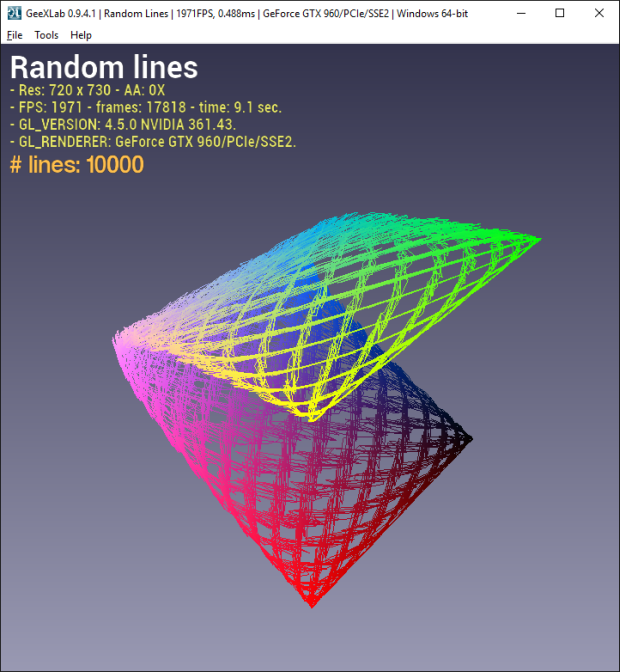INIT script

```local LINE_RENDER_DEFAULT = 0
local LINE_RENDER_STRIP = 1
local LINE_RENDER_LOOP = 2

num_lines = 10000
num_vertices = num_lines + 1

line = gh_polyline.create_v2(num_vertices, LINE_RENDER_STRIP)

math.randomseed(10) -- reproductible random numbers...
local k=0
local i=0

for i=0, nverts-1 do
k = k + 0.1
local t = gxlRandom(-2,2)
local x = math.sin(k*1.1 + t*0.1)
local y = math.sin(k*0.35 + t*0.1)
local z = math.cos(k*0.7 + t*0.1)
gh_polyline.set_vertex_position(line, i, x, y, z, 1)
local r = (x+1) * 0.5
local g = (y+1) * 0.5
local b = (z+1) * 0.5
gh_polyline.set_vertex_color(line, i, r, g, b, 1)
end
```

Where gxlRandom() is the following function:

```function gxlRandom(a, b)
if (a > b) then
local c = b
b = a
a = c
end
local delta = b-a
return (a + math.random()*delta)
end
```

The rendering of the polyline uses more or less the same code than for the simple line:
FRAME script

```gh_camera.bind(camera)

gh_gpu_program.bind(vertex_color_prog)
gh_object.set_scale(line, 10, 10, 10)
gh_object.render(line)
```

The complete demo (gl-210-random-lines.xml) is available in the host_api/Polyline/ folder of the code sample pack.

By modifying the values of the various parameters (k, t, …) you can generate a lot of shapes. The demo comes with 9 built-in shapes: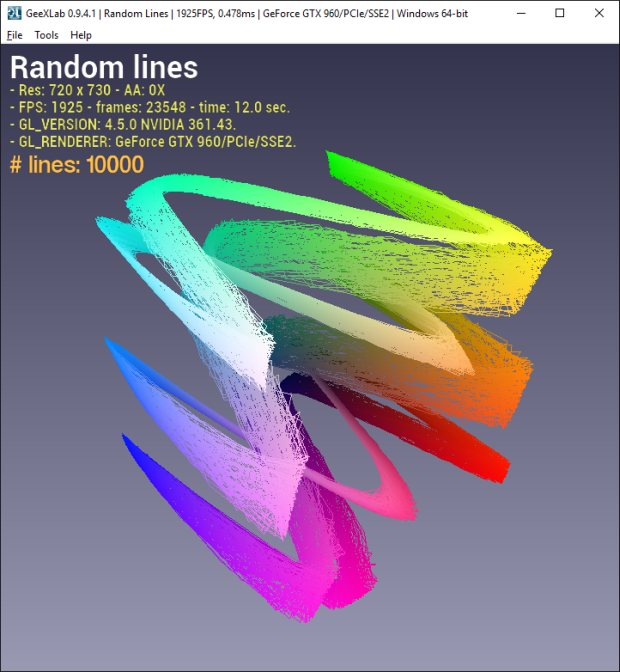Shape 1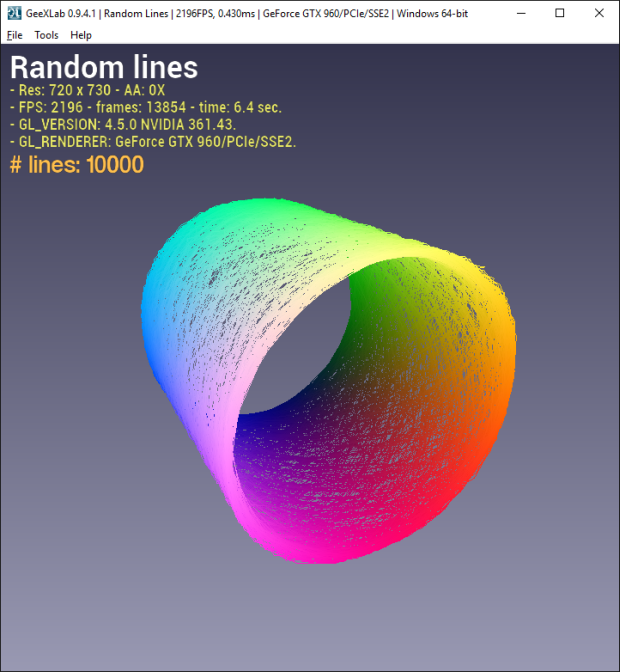Shape 3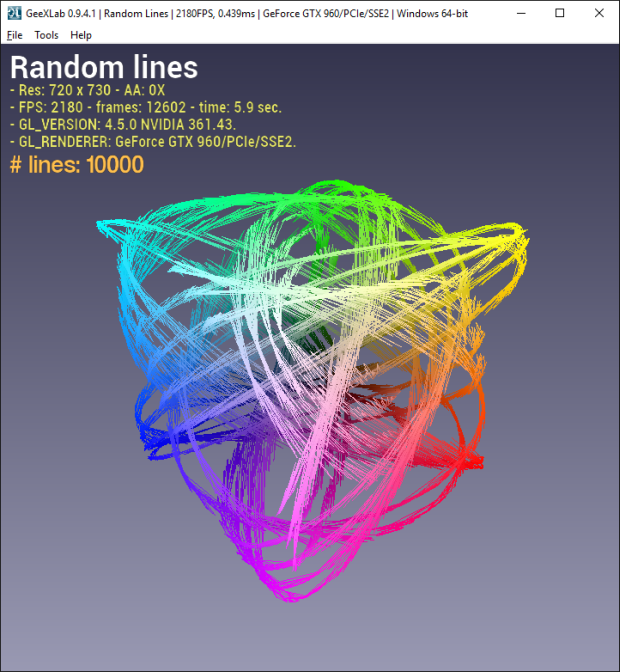Shape 4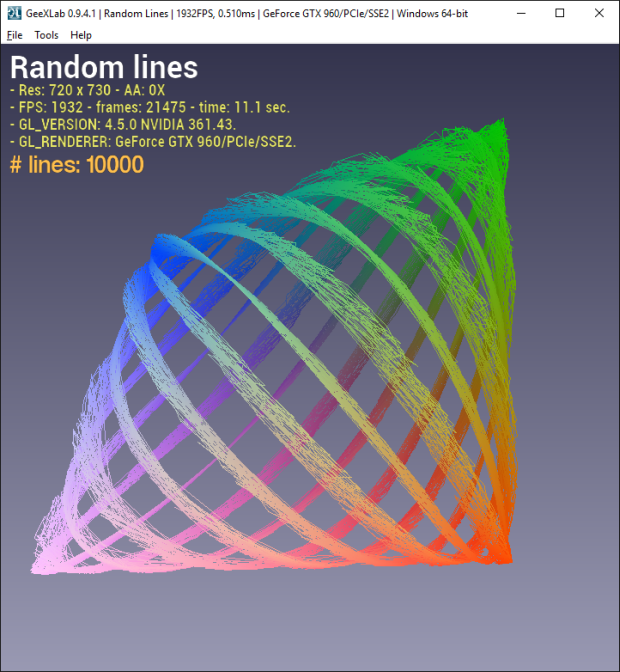Shape 6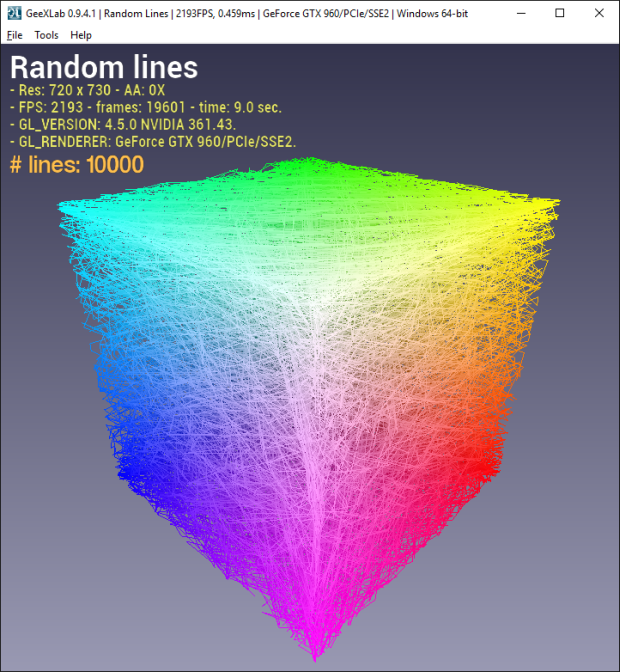Shape 7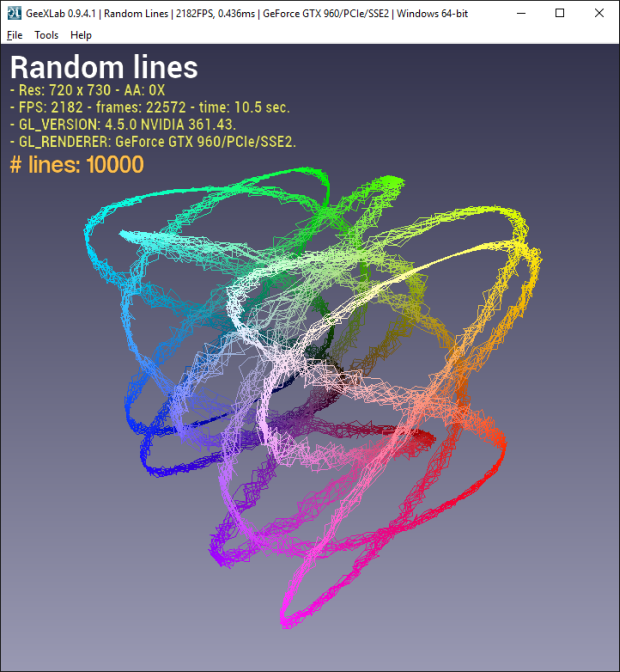Shape 8